American Journal of Theoretical and Applied Statistics
Volume 5, Issue 6, November 2016, Pages: 359-364

Sarima Versus Time Lagged Feedforward Neural Networks in Forecasting Precipitation

Elias Kimani Karuiru1, George Otieno Orwa1, John Mwaniki Kihoro2

1Applied Statistics, Department of Statistics and Actuarial Science, Jomo Kenyatta University of Agriculture and Technology, Nairobi, Kenya

2Computing and E-learning department, Co-operative University College, Nairobi, Kenya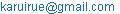(Elias Kimani Karuiru)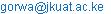(George Otieno Orwa)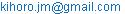(John Mwaniki Kihoro)

Elias Kimani Karuiru, Orwa George Otieno, John Mwaniki Kihoro. SARIMA versus Time Lagged Feedforward Neural Networks in Forecasting Precipitation. American Journal of Theoretical and Applied Statistics.Vol. 5, No. 6, 2016, pp. 359-364. doi: 10.11648/j.ajtas.20160506.15

Received: October 11, 2016; Accepted: October 18, 2016; Published: November 7, 2016

Abstract: The precipitation estimates are considered to be very important in economic planning. Major economic sectors highly depend on the precipitation levels. These sectors include agriculture, tourism, mining and transport. In Kenya, rainfall amount fluctuates with time hence depending on empirical observations while predicting is a hard task. Various statistical techniques have been used in forecasting precipitation. Among these techniques is Holt Winters procedures and SARIMA due to the seasonality effect. SARIMA model has been found to be effective in forecasting precipitation. The model has therefore been the most commonly used while precipitation forecasts are required. However, there is no any statistical research that has been carried out to test the effectiveness of neural networks in forecasting precipitation. This research hence considered forecasting precipitation using SARIMA and TLFN models. Box-Jenkins procedures of forecasting were used. Comparison of forecasts from the two techniques was done through the use of Mean Absolute Deviation (MAD), Mean Squared Deviation (MSD) and Mean Absolute Percentage Error (MAPE) in order to conclude which technique gives the better forecasts. Time Lagged Feed forward Neural Network model performed better than Seasonal Autoregressive Integrated Moving Average

Keywords: Precipitation, Seasonal Autoregressive Integrated Moving Average (SARIMA), Time Lagged Feed forward Neural Network (TLFN), Forecasting

1. Introduction

1.1. Background of the Study

Kenya as a sovereign state depends highly on the agricultural sector. Agriculture has been declared to be the back bone of the Kenyas economy. Other sectors of the economy in Kenya that are dependent on climate include tourism, transport, power generation, mining, and live- stock keeping among others. Also, precipitation forecasts are applied in irrigation and drainage system designs. The performance of these economic sectors depends on the ability of the concerned departments to adapt to climatic fluctuations that have been evident in the recent times. Global warming has been attributed to highly influence the climatic changes in the world. The weather patterns have hence changed significantly resulting into unexpected hardships in implementing development projects. The inability of incorporating climatic changes effect in projects planning stage have highly resulted in their failure or massive government unbudgeted expenditures.

Statistical techniques have been employed in precipitation forecasting. Due to the seasonality effect in precipitation time series, the most commonly used techniques are Seasonal Autoregressive Integrated Moving Average (SARIMA) and Holt Winters forecasting procedures. Each of the technique gives forecasts that take care of trend and seasonality. They have hence been found to be effective in forecasting precipitation. However, there is need to check whether the consideration of neural networks can help in improving the accuracy of the precipitation forecasts. This is due to the already observed capabilities of neural networks. This gives rise to the Time Lagged Feed forward Neural Network (TLFN) model. A comparison of SARIMA and TLFN models forecasts will give rise to the best model that should always be used when fore-casting precipitation, since Holt Winters is a special case of SARIMA model. The selection of the better technique is dependent on its superiority in producing more accurate forecasts. Getting the better technique hence acted as the drive for this study.

1.2. Statement of the Problem

Forecasting precipitation has been done using statistical methods and meteorological approaches. The most commonly used statistical technique is the use of SARIMA model. This is due to trend and seasonality effects in precipitation time series. It is still a statistical problem as to whether consideration of neural networks would improve the precipitation forecasts. This gives rise to consideration of TLFN model in forecasting precipitation. The declaration of the best model is of much help to any interested party when forecasting precipitation. The use of this model will guarantee the most accurate precipitation forecasts possible.

1.3. Justification of the Study

The main aim of this project was to compare the forecasting performance of SARIMA and TLFN models in forecasting precipitation. The conclusion of the project gives the method that should be applied by default when forecasting precipitation.  The application of the said method hence guarantees most accurate forecasts possible. Decision making process is hence be hastened hence saving time.

1.4.Objectives

1.4.1.General Objective

To compare the performance of SARIMA and TLFN models in forecasting precipitation.

1.4.2. Specific Objective

1.  To identify the order of SARIMA model that fits the precipitation time series data.

2.  To use the SARIMA model identified above to forecast precipitation.

3.  To identify the order of TLFN model that fits the precipitation time series data most accurately.

4.  To use the TLFN model identified above in (3) to forecast precipitation.

2. Review of the Previous Studies

Forecasting is the process of predicting future events. Forecasting hence forms the underlying basis of all business decisions. The forecaster may never get it right, but may always get it less wrong. Forecasts are hence seldom perfect. Most forecasting methods assume that there is some underlying stability in the system and future will be like the past (Jani, 2014). However, the accuracy of the forecasts decreases with length of forecasts. Qualitative forecasting methods are subjective in nature since they rely on human judgment and opinion (Satya et al., 2007). They are hence normally used when situation is vague and little data exist. Quantitative forecasting methods use mathematical or simulation models based on historical demand or relationships between variables (Chatfield,2000). They involve mathematical techniques which include smoothing, trend projection and trend projection adjusted for seasonal influence. Each of the technique suits a given situation and hence there is need to clearly understand the time series before deciding on which technique to apply. The existing computers of tare automated to undertake the forecasting procedure make site a sy to identify the best technique suiting the situation at hand.

Global warming has made rainfall prediction to be a challenging task in the modern world .Its effect has made the previously employed methods ineffective. Earlier forecasting methods such as simple quantitative precipitation forecasts used by Klein and Lewis (1970), Glahnand Lowry (1972) and Pankratz (1983) have lost their edge due to the changing patterns and variability in rainfall. Borlando et al. (1996) used ARIMA models to forecast hourly precipitation in the time of their fall and the amounts obtained were compared with the data to measure rain.

They came to the conclusion that with increasing duration of rainfall, the predictions were more accurate, and shorter duration of rainfall, rain rate difference will be more than the actual corresponding value. Kane and Yusof (2012) analyzed the precipitation forecast using SARIMA model in Golastan province and found the seasonality measure in SARIMA to be highly useful in modeling precipitation. Kibunja et al.(2014) studied the effectiveness of SARIMA model in forecasting precipitation in Mount Kenya region and concluded that the model was good. Sopipan (2014) forecasted rainfall in Thailand using SARIMA and Holt Winters models and found both models to be adequate.

Artificial Neural Network (ANN) models have been used to predict atmospheric variables including precipitation. Hall et al.(1999) used ANNs to predict precipitation for a 24 hour period. Their ANN model had a Brier score (Brier, 1950) of 0.25 and a Briers kill score (Wilks, 1995) of 0.73 using 0.342 as the reference score. Kuligowski and Barros(1998) had mixed results when comparing the use of ANNs to linear regression for quantitative precipitation forecasts for a 24-hour prediction period. In comparison to linear regression, they found that the ANN model had a lower threat score (Schaefar,1990) when the observed precipitate on amount was below 15 mm and a higher threat score when the observed amount was above15mm. A threat score measures the accuracy of positive forecasts and ranges from zero to one with one being a perfect score. Using spatial and temporal data of recent rainfall, Luk et al. (2000) developed an ANN model for predicting flash flood rainfall amounts for 15 minutes ahead for several areas of western Sydney, Australia. Recently, Time Lagged Feed forward Networks, another Artificial Neural Network algorithm have successfully been used with different hydrologic modeling studies. Results from different studies suggest an encouraging performance by TLFN algorithm in comparison to other statistical models. Keeping this in view the better performance of TLFN for different studies, this study was designed to evaluate the performance of the TLFN in comparison to SARIMA in predicting of monthly rainfall values in Kenya.

3. Research Methodology

In this section, we discuss SARIMA model. We then discuss Time Lagged Feed Forward Neural Network. Lastly, we discuss the model performance measures.

3.1. SARIMA Model

Autoregressive Process (AR)

A stochastic process yt is said to be an AR process of order p, denoted by AR (p), if

yt=φ1yt−1+φ2yt−2+...+φpyt−p+εt

The AR(p) process value is obtained through regressing the past pown values then adding a random hock εt.

Moving Average Process (MA)

A stochastic process yt is said to be an MA of order q, denoted as MA(q),if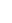yt0εt1εt1+...+θqεtq

The MA(q) process value is obtained through regressing own past errors

Autoregressive Moving Average Process (ARMA)

ARMA process is made up of AR(p) and MA(q)processes. A stochastic process yt is said to be an ARMA process of order p,q, denoted as ARMA(p,q),

yt=εt+(φ1Yt1+φ2Yt2+...+φpYtp)+(θ0εt1εt1+...+θqεtq).

This process can be re-framed through the use of back-shift operator B. The re-framed process hence become,

a(B)Yt=b(B)εt

Where a(B)=1+φ1B+...+φpBp and Bb(B)=1+θ1B+...+θqBq

Autoregressive Integrated Moving Average Process (ARIMA)

The ARMA process broadening to accommodate differencing gave rise to the ARIMA process. A stochastic process yt is said to be an ARIMA process with order p, d, q denoted as ARIMA (p, d, q), if (1−B)d Yt is a casual ARMA (p,q), then the corresponding ARIMA process become, a(B)(1−B)dYt=bq(B)εt

Seasonal Autoregressive Integrated Moving Average process (SARIMA)

Box-Jenkins introduced the SARIMA process that takes care of non-stationery time series con- training seasonality. A stochastic process is defined as,

φp(B)ΦP(Bs)(1−B)d(1−Bs)Dytq(B)ΘQ(Bs)εt

Where, φp(B),,ΦP(Bs),θq(B) and Θ Q(Bs)are the characteristic polynomials of orders p, P, q, and Q respectively. D is the order of non-seasonal differentiated and D is the order of seasonal differencing.

3.2. Time Lagged Feed Forward Network

Feed forward neural networks are widely used for forecasting financial data due to its ability to predict the dependent samples (Jose et al., 2000). It is an extension of the multi layer perception that includes nonlinear processing elements in a feed forward design. The time lagged feed forward neural network (TLFN) is a dynamic network with short-term memory elements provided at the input layer in the

Form tap-delay lines giving the network ability to store past data (Jose etal., 2000).  The depth of the short-term memory is one plus the number of delays and the resolution of the network is one. The tap delay lines with their corresponding weights connecting to the first hidden layer constitute impulse response filters in linear combiners. Following these temporal components is the typical nonlinear static MLP. Thereafter, the MLP processes the present and past values of the network input layers.

The diagram below is an example of TLFN.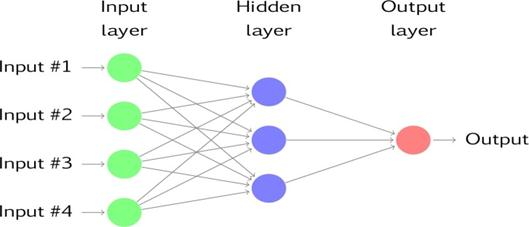Figure 1. Time lagged feed forward Network example.

Given an input signal consisting of the present value x(n) and the past values x(n−1),...,x(n− p) stored in a delay line memory of order p, the free parameters of the network are adjusted to minimize the mean-squared error between the output of the network and the desired(or target) response. At time n, the temporal pattern applied to the input layer of the network is the signal vector:

x(n)=[x(n),x(n−1),...,x(n−p)]

which may be viewed as a description of the state of the non linear filter at time n. Considering an input  vector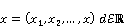,

and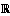is the real line, the input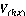to the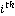hidden node is the value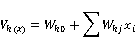and the output becomes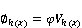3.3. Model Performance Measures

There are various statistics that can be used to compare different forecasting methods. These statistics include MAPE, MAD, and MSD. Although these statistics are not much informative, smaller values usually indicate a better fitting model.

3.4. Area of Study

This study concentrated on developing statistical models of precipitation in Kenya. Performance of SARIMA and TLFN models in forecasting precipitation was compared to determine the best method.

3.5. Description of the Data

The data that was used in this study comprised of precipitation monthly values from 1990-2012. Data from 1990-2010 was used in model identification while the data in the remaining two years was used in determining the best model in forecasting between the four models. The data was obtained from meteorological department.

4. Results and Discussion

4.1. SARIMA Model

SARIMA modeling requires the stationarity condition to be satisfied. Augmented Dickey Fuller (ADF) test was used to test for it. The p-value obtained from the ADF test is 0.01.We therefore failed to accept the null hypothesis at 5% level of significance and concluded that the data is stationary. There hence no need for differencing.

The best model was selected by comparing the AIC value. Model (1, 0, 0) (1, 1, 1)12 was selected as the most appropriate model since it has the least values of the information criterions. This model has two significant variables. The correlation matrix of this model was examined. The correlation between the parameters of the model was a weaker one. This implies that all the parameters are important in fitting the model.

4.1.1. Diagnostic Checking

The residuals were checked to find out if they followed a white noise process. This was achieved by plotting the residual Q-Q and normality test plots as shown in figure 2. The Q-Q plot is reasonably straights on normality is okay.

In addition, the ACF plot of the residuals in figure3 shows that for the first 20 lags, all sample autocorrelations fall inside the 95% confidence bounds indicating the residuals appear random.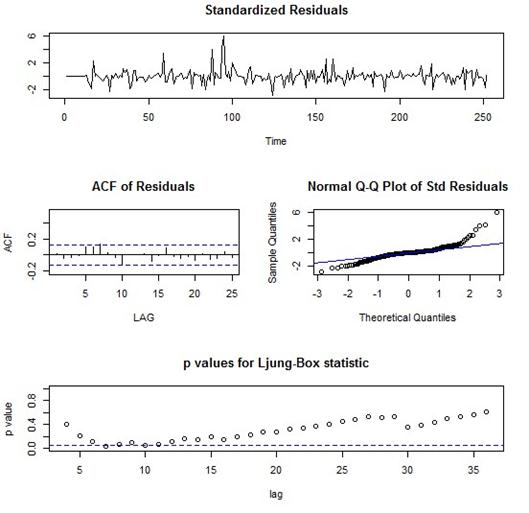Figure 2. Diagnostic checking.

4.1.2. Forecasting

The main aim of this study was to formulate a SARIMA model that fits our precipitation data and use the model to forecast. Having identified SARIMA (1,0,0)(1,1,1)12 as the most appropriate SARIMA model, we used the model to forecast the next 24 monthly precipitation values. To test the capacity of the model to forecast, the forecasts were compared with the real values and MAPE, MAD and MSD performance statistics computed. Figure 3 shows a graph of forecasts while the performance statistics are shown in Table 1.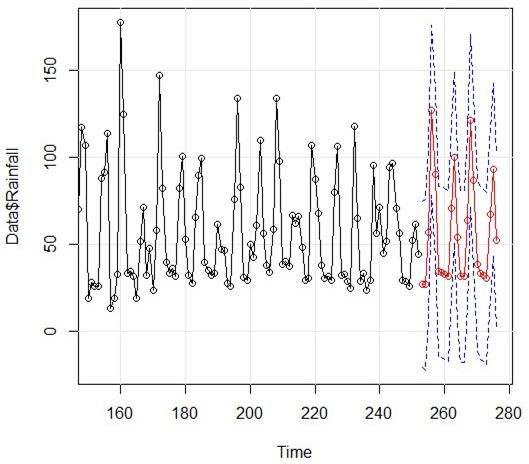Figure 3. Plot of forecasts using SARIMA model.

Table 1. Mean squared deviation, mean absolute deviation and mean absolute percentage error of SARIMA.

 SARIMA MAPE 21.01317 MSD 272.2866 MAD 10.56939

4.2. Time Lagged Feed Forward Neural Network

To find the optimal number of hidden nodes and time lags we trained the network with different values of above parameters until the mean square error of training set is very minimum, and check the testing set performance. For whichever values of above parameters, the mean square errors training set and testing set is minimum.  We changed the different step sizes (learning rates) and momentum rates, but we have observed that, by taking the different learning rates in hidden layer and output layer with a constant momentum rate leads to fast training. By changing the mean and range of the weights in hidden synapse and output synapse, we can obtain a good performance of testing set. When the model is generalized, then the parameters of the network are chosen as optimal values of the different parameters. To train and test the network, we used R software.

We developed and trained several time lagged feed forward neural networks models for forecasting monthly rainfall.  The best models observed from the several TLFN model is 12-6-1. The input layer has 12 time lags; the hidden layer has 6 hidden nodes and the output layer have one node. Figure 4 shows a graph of Forecasts using the TLFN model.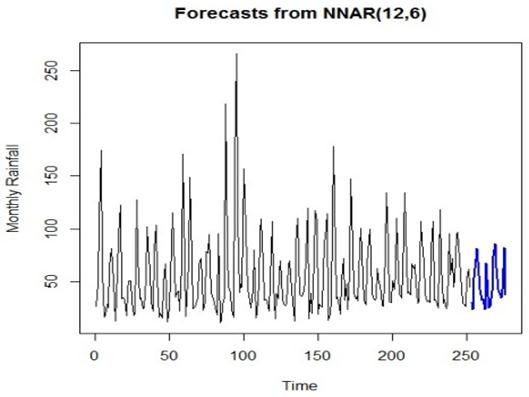Figure 4. Forecasts using TLFN model.

Upon testing the model, it exhibited the following statistics in table 2.

Table 2. Mean squared deviation, mean absolute deviation and mean absolute percentage error of TLFN.

 TLFN MAPE 8.23406 MSD 52.46044 MAD 4.324458

4.3. Comparison of Forecasts

The mean squared deviation (MSD), mean absolute deviation (MAD) and mean absolute percentage error (MAPE) of SARIMA and TLFN models are given in table 3.

Table 3. Comparison of SARIMA and TLFN.

 Measurement of forecasting error SARIMA TLFN MAPE 21.01317 8.23406 MSD 272.2866 52.46044 MAD 10.56039 4.324458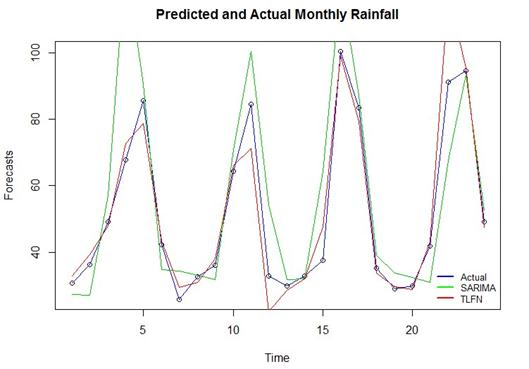Figure 5. Graph of forecasts from SARIMA and TLFN in comparison with the actual values.

5. Conclusion

It is very clear that neural network model is the best to forecast the future values of precipitation. This is because it has the minimum measures of MAPE, MAD and MSD. TLFN also produced R-squared value of 0.9218805 as compared to 0.7176565 of SARIMA model. We therefore concluded that forecasting of precipitation with Time Lagged Feed forward Neural Network is more efficient than that of SARIMA model.

Neural networks therefore present an alternative approach that is promising to time series fore- casting. The forecasting performance of neural networks is highly dependent on the network structure, and Training procedure. However, the difficulty in identifying good raw data, preprocessing the data, training the network and repeating this process until a good model is developed should not be discounted.

The lack of systematic procedures for Neural network forecasting models building is hindering the exploitation of full potential of the models. Their applicability is hence being hindered by lack of systematic procedures and good theoretical background.

References

1. Borlando, P, R Montana and Raze (1996), ‘Forecasting hourly precipitation in time of fall using arima models’, Journal of Atmospheric Research 42(1), 199–216.
2. Box, G E and G M Jenkins (1970), Time Series Analysis: Forecasting and Control, Holden-Day, San Franciscoi.Heinz, G, L J Peterson, R W Johnson and Kerk C J (2003), ‘Exploring relationships in bodydimensions’, Journal of Statistics Education 11, 1–15.
3. Brier, G .W (1950), ‘Verification of forecasts expressed in terms of probability.’, MonthlyWeather Review 78(1), 1–3.
4. Chatfield, C (2000), Time-Series Forecasting, Chapman and Hall CRC, New York.
5. Glahn, Harry and Dale A Lowry (1972), ‘The use of model output statistics mos in objective weather forecasting’, Journal of Applied Meteorology 11, 1203–1211.
6. Hall, Brooks and Doswel (1999), ‘Precipitation forecasting using a neural network. weather and forecasting’, Journal of Applied Meteorology 14(3), 338–345.
7. Jani, P (2014), Business Statistics:Theories and Applications, PHI Learning Private Limitedl, Delhi.
8. Jose, Neil R Euliano and W. Curt Lefeb (2000), ‘Neural and adaptive systems: Fundamentals through simulations’, Journal of Applied Time series Analysis .
9. Kane, Ibrahim Lawal and Fadhilah Yusof (2012), ‘Modelling monthlyrainfall time series using ets and sarima’, International Journal of Current Research 4(1), 195–200.
10. Kevin, Swingler (1996), ‘Applying neural networks: A practical guide’.
11. Kibunja, Hellen, George Orwa and John Kihoro (2014), ‘Forecasting precipitation in mt kenya’,Journal of Applied Time series Analysis 7(2), 87–105.
12. Klein, William H and Frank Lewis (1970), ‘Computer forecasts of maximum and minimum temperature’, Journal of Applied Meteorology 9, 350–359.
13. Kuligowski and Barros (1998), ‘Localized precipitation forecasts from a numerical weather prediction model using artificial neural networks.’, Weather and Forecasting 13(4), 1194–1204.
14. Luk, K.C, Ball and Sharma (2000), ‘A study of optimal lag and spatial inputs for artificial neural network for rainfall forecasting.’, Journal of Hydrology 227, 56–65.
15. Oliver, Anderson D (1977), ‘Time series analysis and forecasting: Another look at the box- jenkins approach’, Journal of Royal Statistical Society 26(4), 285–353.
16. Pankratz, A (1983), Forecasting with Univariate Box-Jenkins Models: Concepts and Cases, John Wiley and Sons, New Yorki.
17. Russell, Reed and Robert J Marks II (1998), ‘Neural smithing: Supervised learning in feedfor- ward artificial neural networks’, Journal of Applied Time series Analysis 7(2).
18. Satya, P, V Ramasubramanian and S C Menta (2007), ‘Statistical models for forecasting milk production in india’, Journal of the Indian Society of Agricultural Statistics 6, 80–83.
19. Schaefar, J.T (1990), ‘The critical success index as an indicator of warning skill.’, WeatherForecasting 5(4), 570–575.
20. Sopipan, N (2014), ‘Forecasting rainfall in thailand: A case study of nakhon ratchasima province’, International Journal of Environmental, Chemical, Ecological, Geological and Geographical Engineering 8(11).
21. Wilks, D.S (1995), ‘Statistical methods in the atmospheric sciences’, Journal of Applied Mete- orology p. 467.

 Contents 1. 1.1. 1.2. 1.3. 1.4. 2. 3. 3.1. 3.2. 3.3. 3.4. 3.5. 4. 4.1. 4.2. 4.3. 5.
Article ToolsAbstractPDF(320K)